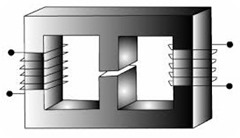# Laws of the Magnetic Circuit# Laws of the Magnetic Circuit

It stated that the laws governing the steady flow of electricity in a circuit may readily be modified. So as to be at once applicable to the magnetic circuit. Thus
Magnetomotive Motive Force = Flux x Reluctance
F= Φ x S

Corresponding exactly to Electromotive Force = Current x Resistance
Reluctance = (length/area) x (1/Permeability)
= l/Aμ

For a magnetic of uniform sectional area corresponding exactly to
Resistance = (length/area) x (1/conductivity)

It often convenient to calculate in terms of unit dimensions. We then have
m.m.f per unit length = Flux x Reluctance per unit length
= (Flux/area) x (l/permeability)
= Flux density x (l/permeability)
Magnetic Field Intensity H = B/μ
This corresponds exactly to,
For a material carrying a uniform distributed flux and of length l, then the total m.m.f is then equal to the m.m.f per unit length x l
i.e. F=Hl or AT=Hl

For dealing with magnetic circuit in which the flux has to permeate a number of different portions in seconds, the methods employed for dealing with series electric circuits can at once be applied, the total reluctance being the sum of the values for the various portions. Normally, the values of the reluctance are only of moment in so far as it permits of a value being determined for the m.m.f required to establish a given flux in the circuit. It is often the simplest method to determine this value of the total m.m.f by summing the values of m.m.f necessary to establish the flux through the various component parts of the circuit. This is exactly in line with the method of calculating the total voltage drop in an electric circuit by summing the values of the voltage drop in the various parts.

Thus the total value of the m.m.f facing around a complete magnetic is given by
AT (or) F = Hdl

Or where the circuit consists of a number of a homogeneous parts, each of uniform cross section, and length l1, l2 etc.

Total m.m.f            F (or) AT = Σ Hl = H111 + H2l2 + ….
= Φ [S1 + S2 + ….]

If the values for the area and permeability of the various portions of the circuits are, respectively, A1, μ1, etc., the total m.m.f becomes
AT (or) F = (B11)l1 + (B22)l2 + …..
Where B1 = Φ/A1 etc

Occasionally it is convenient to express the fundamental law of the magnetic circuit in the form.
Flux = magnetomotive force x Permeance

Permeance is nothing but the reciprocal of the reluctance, for dealing with paths in parallel, the total Permeance is the sum of the values for the individual paths.

The main difference between electric and magnetic calculations arises from the fact that whereas the resistance of an electric circuit is not directly dependent upon the values of the reluctance of a magnetic substance is dependent to an enormous extent upon the value of the flux permeating it.0 CommentsComments